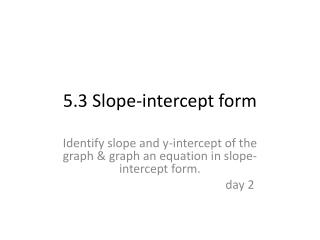DownloadDownload Presentation5.3 Slope-intercept form

# 5.3 Slope-intercept form

Download Presentation## 5.3 Slope-intercept form

- - - - - - - - - - - - - - - - - - - - - - - - - - - E N D - - - - - - - - - - - - - - - - - - - - - - - - - - -
##### Presentation Transcript

1. 5.3 Slope-intercept form Identify slope and y-intercept of the graph & graph an equation in slope-intercept form. day 2

2. Write an equation of the line in slope-intercept form that passes through the points (2,1) and (5,-8). y = mx + b (x1, y1) (x2, y2) y = -3x + 7 m = ___-___ - -8 1 = -9 = -3 m = b = -3 2 3 5 7 x = y = 2 Solve for b: 1 y = mx + b 1= -3( 2) + b 1= -6+ b 7= b

3. Write an equation of the line in slope-intercept form that passes through the points (-3,4) and (2,-1). y = mx + b (x1, y1) (x2, y2) y = -1x + 1 m = ___-___ - -1 4 = -5 = -1 m = b = -1 -3 5 2 1 x = y = -3 Solve for b: 4 y = mx + b 4= -1( -3) + b 4= 3+ b 1= b

4. Steps to graph an equation in slope-intercept form. y = mx + b y = ¾x + 1 • Identify m and b • m = • b = ¾ 1 2. Put the y-intercept (b) on the y-axis. • Use your slope to get to the next point. Rise ---if positive: go up; if negative: go down Run--- go to the right 4. Connect the points.

5. Steps to graph an equation in slope-intercept form. y = mx + b y = 3x • Identify m and b • m = • b = 3 1 3= 0 2. Put the y-intercept (b) on the y-axis. • Use your slope to get to the next point. Rise ---if positive: go up; if negative: go down Run--- go to the right 4. Connect the points.

6. Steps to graph an equation in slope-intercept form. y = mx + b y = 4 • Identify m and b • m = • b = 0 4 2. Put the y-intercept (b) on the y-axis. • Use your slope to get to the next point. Rise ---if positive: go up; if negative: go down Run--- go to the right 4. Connect the points.

7. Write an equation in slope-intercept form. y = mx + b • Identify m and b • m = • b = -1 4 y = -1x + 4 Or y = -x + 4

8. Assignment: page 312: 22-34,37-39, 48,49 Note: 27: will have a decimal for the y-intercept